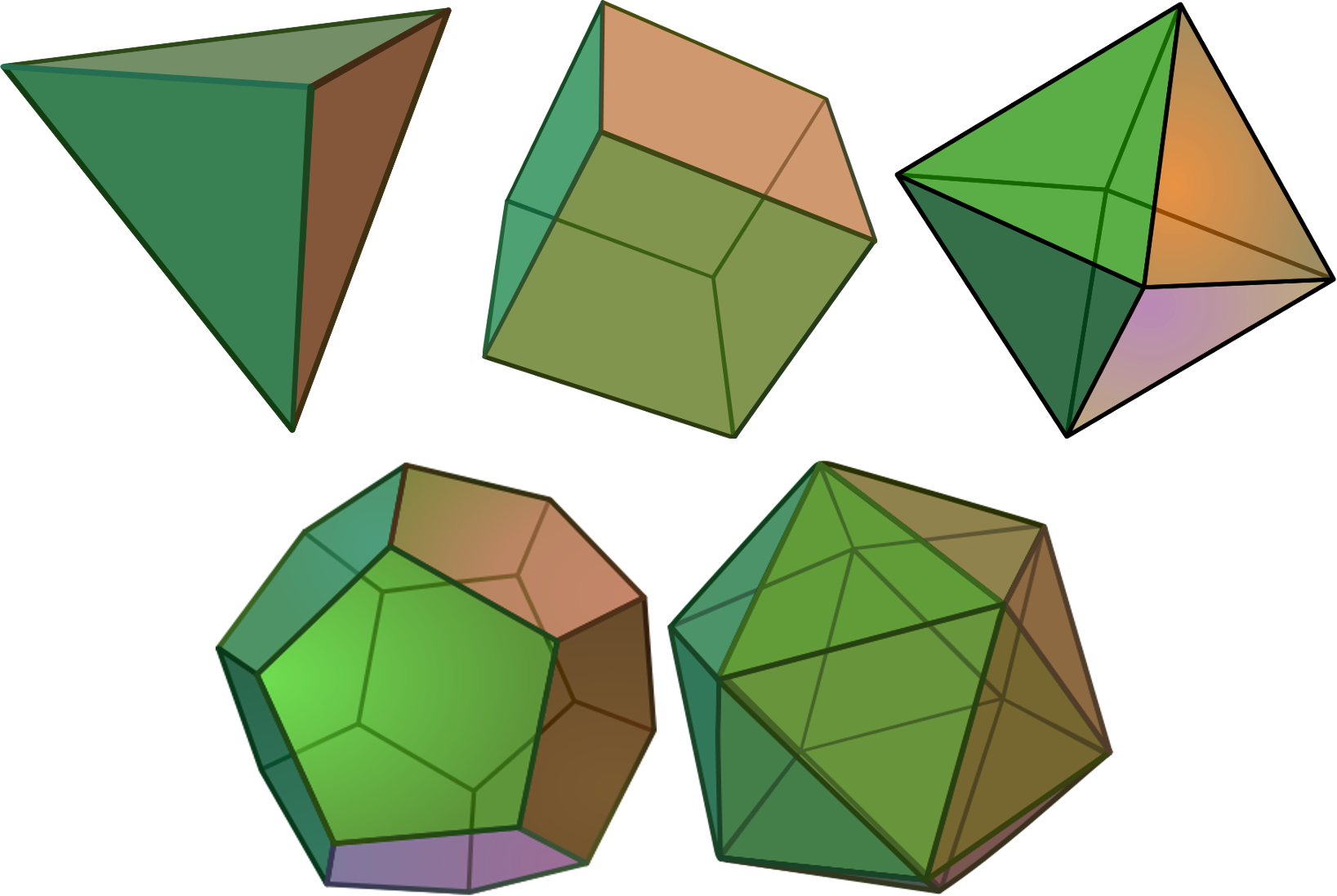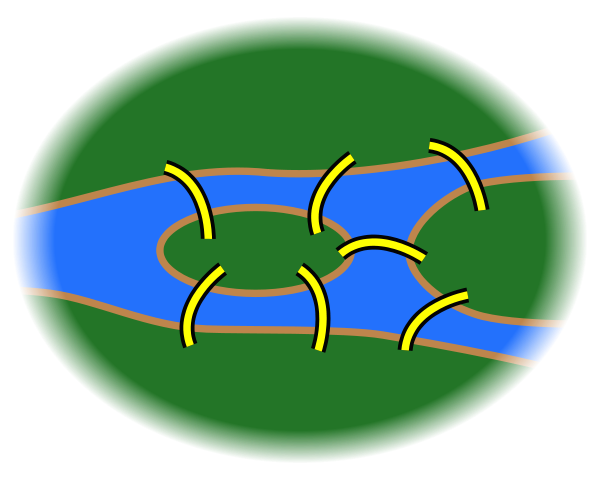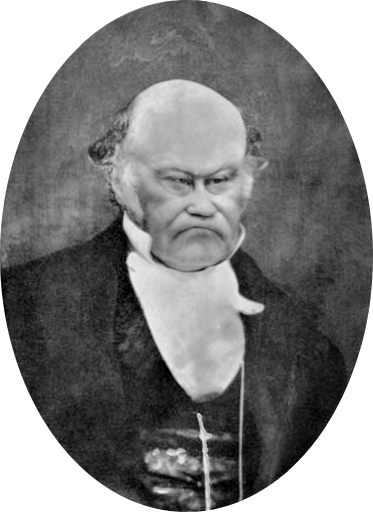# The Unintentionally Useful Consequences of Playing Games with Maths

Christian Lawson-Perfect## Unintentionally useful maths I won't be talking about today

• Found the Fibonacci numbers in my baby's stacking cups
• Used error-correcting codes to memorise a lullaby
• Wrote an algorithm for finding graph automorphisms while extending a puzzle

## The unintentionally useful maths I will be talking about today

One day, I was playing about with a remarkably mathematical shape.

## Platonic solids

A polyhedron is a 3-dimensional shape with flat sides and straight edges.

A Platonic solid is a solid whose faces are all the same regular polygon, with the same number of faces meeting at each vertex.## What can we say about a polyhedron?• Number of edges, faces, sides.
• Shapes of the faces.
• Symmetries.

## Graphs

A graph is a collection of points (vertices), and the edges joining them.

It doesn't matter where the points are, or what shape the edges are, or if the edges cross.

## What can we say about a graph?

• How many vertices and edges?
• How many edges connecting each vertex?
• Does it look like a hedgehog?

## What can we say about a graph?

Can you colour the vertices so that no two neighbours have the same colour?

OR

Can you arrange the vertices on two sides of a line so that no edge stays on the same side of the line?

A graph with this property is called bipartite.

## What can we say about a graph?

Can you draw a path which goes along every edge once, and ends where it started?

A graph with this property is called Eulerian.

## The bridges of Königsberg## What can we say about a graph?

Can you draw a path which goes through each vertex exactly once, and ends where it started?

A graph with this property is called Hamiltonian.

## A Theorem

Every bipartite graph with an odd number of vertices is
non-Hamiltonian.## The Herschel graph

A colleague showed me the Herschel graph, and asked what I could do with it.

The Herschel graph is the smallest non-Hamiltonian polyhedral graph.

Polyhedral?

## Unintentionally useful maths

Graphs, groups and polyhedra turn up just about everywhere!